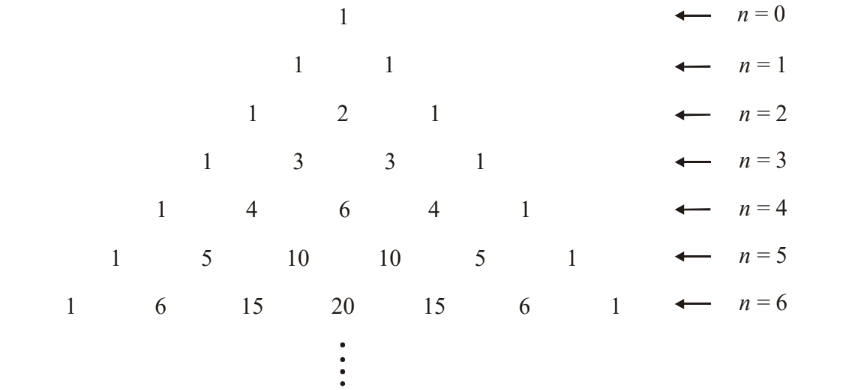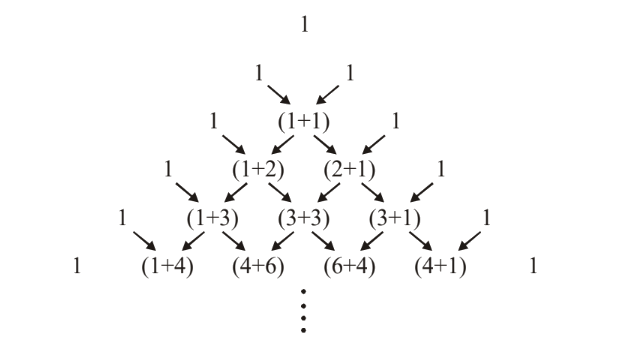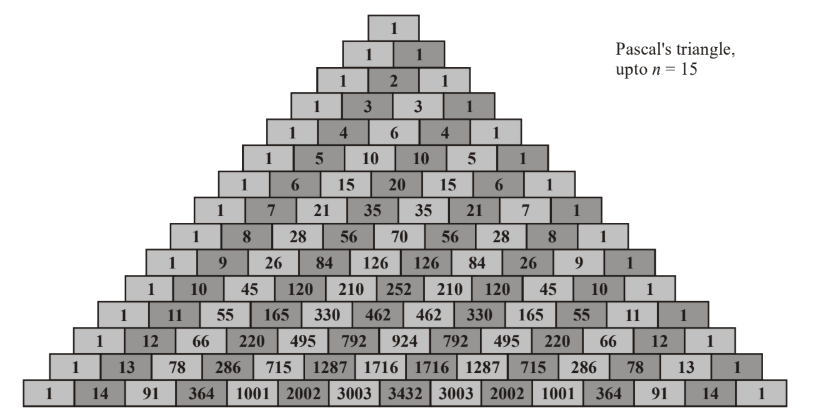# Introduction To Binomial Theorem

Go back to  'Binomial Theorem'

Binomial theorem is something that has been known to mathematicians since many centuries ago. In this introduction, we’ll trace the origins of this theorem to the coefficients we obtain when we expand any binomial term raised to an integral power.

Consider the following expansions, which can be verified by direct multiplication:

$\begin{array}{l}{\left( {x + y} \right)^0} \qquad = \qquad 1 & \\{\left( {x + y} \right)^1} \qquad = \qquad x + y\\{\left( {x + y} \right)^2} \qquad = \qquad {x^2} + 2xy + {y^2}\\{\left( {x + y} \right)^3} \qquad = \qquad {x^3} + \,3{x^2}y\,\, + \,3x{y^2} + {y^3}\,\\{\left( {x + y} \right)^4} \qquad = \qquad {x^4} + \,\,4{x^3}y\,\, + \,6{x^2}{y^2} + 4x{y^3} + {y^4}\,\\{\left( {x + y} \right)^5} \qquad = \qquad {x^5} + \,5{x^4}y\,\, + \,10{x^3}{y^2} + 10{x^2}{y^3} + 5x{y^4} + {y^5}\\{\left( {x + y} \right)^6} \qquad = \qquad {x^6} + \,6{x^5}y\,\, + \,15{x^4}{y^2} + 20{x^3}{y^3} + 15{x^2}{y^4} + 6x{y^5} + {y^6}\\\,\,\,\,\,\,\,\,\,\,\,\,\,\,\,\,\,\,\,\,\,\,\,\,\,\,\,\,\,\,\,\,\,\,\,\,\,\,\,\,\,\,\,\,\,\,\,\,\,\,\,\,\,\,\,\,\,\,\,\,\,\,\,\,\,\,\,\,\,\,\,\,\,\,\,\,\,\, \vdots \,\end{array}$

and so on

Do you notice anything special about these expansions, in particular, any general rule or trend these expansions follow that might enable us to expand $${\left( {x + y} \right)^n}$$ directly for a general n? First of all, notice that the number of terms in each expansion is one more than the power of the binomial term. For example, $${\left( {x + y} \right)^5}$$ has 6 terms.

However, mathematicians long back also realized another important fact, namely, the relation between the coefficients obtained upon expansion. To see what this relation is, let us write the coefficients in the following ‘triangular’ pattern:Fig. 20

Do you observe any relation between the various coefficients. If not, consider this same arrangement in a slightly modified form:Fig. 21

The ‘rule’ for constructing this triangular pattern should be pretty obvious now. All edge-numbers are 1. Any other is obtained by adding the number directly above and to the left with the number directly above and to the right, as in Fig-34 Extending this process gives us all the ‘binomial coefficients.’ This geometrical arrangement of the binomial coefficients in a triangle is called Pascal’s triangle. The figure below shows a Pascal triangle containing the coefficients upto n = 15.Fig. 22

If we denote the (i + 1) th  number at the n th  level by T n, i , then we have

${T_{n,\,\,i + 1}} = {T_{n - 1,\,i}} + {T_{n - 1,\,\,i + \,1}}\,\,\,\,\,\,\,\,\,\,\,\,\,\,\,\,\,\,\,\,\,\,\,\,\,\,\,\,\,...{\rm{ }}\left( 1 \right)$

Later on, when we actually write  in terms of combinational notation (in fact, we’ll see that $${T_{n,\,\,i + 1}}$$   corresponds to $$^n{C_i}$$ ), we will immediately understand that (1) is equivalent to

$^n{C_i} = {\,^{n - 1}}{C_{i - 1}} + {\,^{n - 1}}{C_i}$

which, as we already know from the last chapter on P & C, is true.